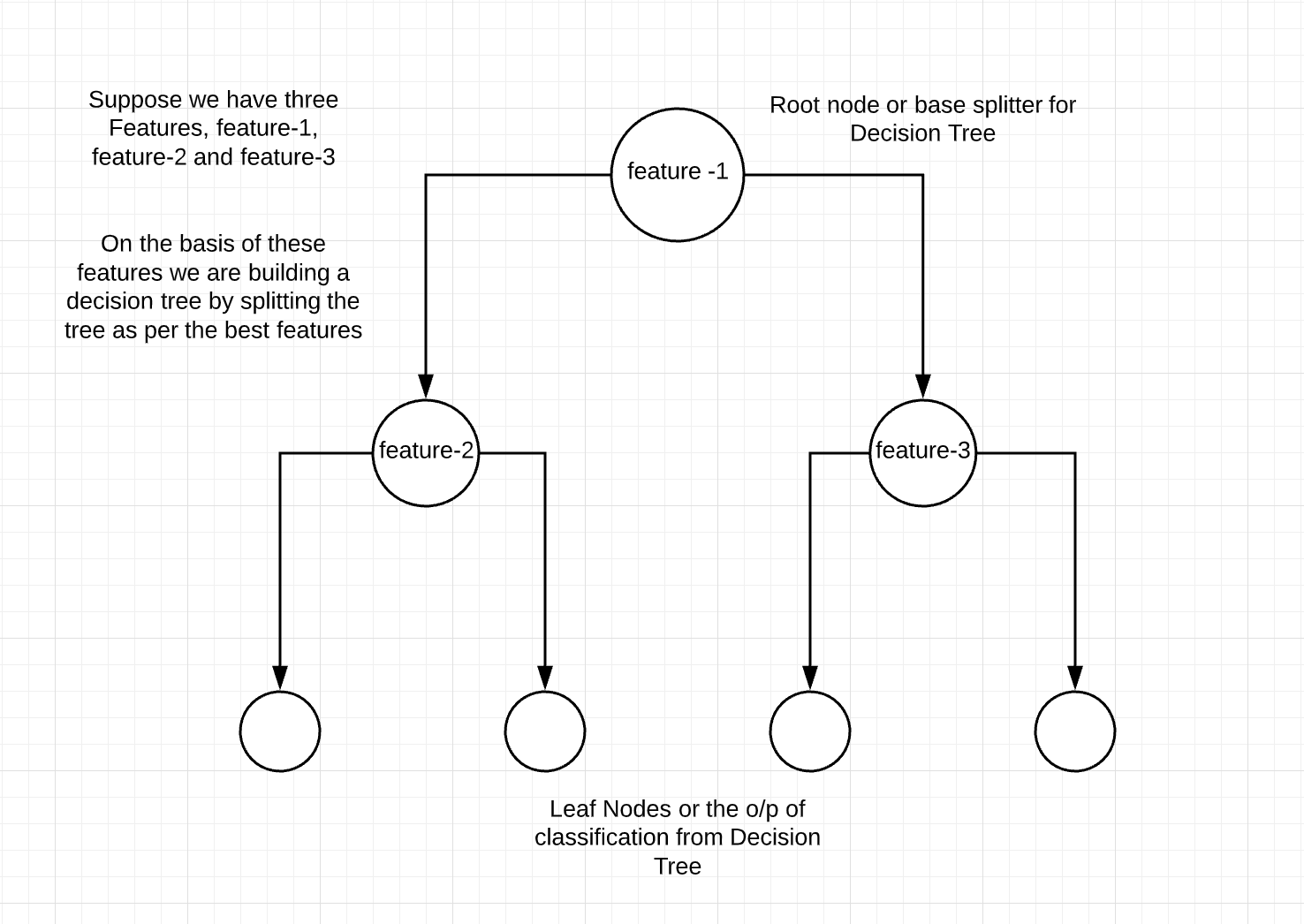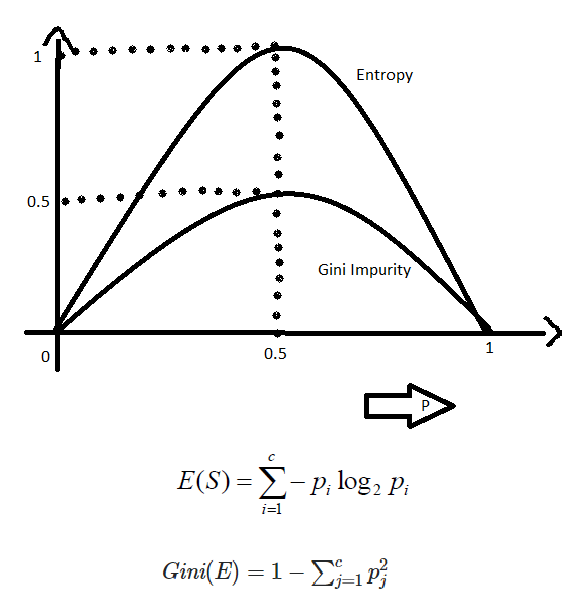Related Articles

# ML | Gini Impurity and Entropy in Decision Tree

• Difficulty Level : Easy
• Last Updated : 08 Sep, 2021

Machine Learning is a Computer Science domain that provides the ability for computers to learn without being explicitly programmed. Machine Learning is one of the most highly demanded technology that everybody wants to learn and most of the companies require highly skilled Machine Learning engineers. In this domain, there are various machine learning algorithms developed to solve complex problems with ease. These algorithms are highly automated and self-modifying, as they continue to improve over time with the addition of an increased amount of data and with minimum human intervention required. To learn about top Machine Learning algorithms that every ML engineer should know click here

In this article, we will be focusing more on Gini Impurity and Entropy methods in the Decision Tree algorithm and which is better among them.Decision Tree is one of the most popular and powerful classification algorithms that we use in machine learning. The decision tree from the name itself signifies that it is used for making decisions from the given dataset. The concept behind the decision tree is that it helps to select appropriate features for splitting the tree into subparts and the algorithm used behind the splitting is ID3. If the decision tree build is appropriate then the depth of the tree will be less or else depth will be more. To build the decision tree in an efficient way we use the concept of Entropy. To learn more about the Decision Tree click here. In this article, we will be more focused on the difference between Gini Impurity and Entropy.

Entropy:
As discussed above entropy helps us to build an appropriate decision tree for selecting the best splitter. Entropy can be defined as a measure of the purity of the sub split. Entropy always lies between 0 to 1. The entropy of any split can be calculated by this formula.The algorithm calculates the entropy of each feature after every split and as the splitting continues on, it selects the best feature and starts splitting according to it. For a detailed calculation of entropy with an example, you can refer to this article

Gini Impurity:
The internal working of Gini impurity is also somewhat similar to the working of entropy in the Decision Tree. In the Decision Tree algorithm, both are used for building the tree by splitting as per the appropriate features but there is quite a difference in the computation of both the methods. Gini Impurity of features after splitting can be calculated by using this formula.For the detailed computation of the Gini Impurity with examples, you can refer to this article. By using the above formula gini Impurity of feature/split is being calculated.

Entropy v/s Gini Impurity:
Now we have learned about Gini Impurity and Entropy and how it actually works. Also, we have seen how we can calculate Gini Impurity/Entropy for a split/feature. But the major question arises here is why do we need to have both the methods for computation and which is better?The internal working of both methods is very similar and both are used for computing the feature/split after every new splitting. But if we compare both the methods then Gini Impurity is more efficient than entropy in terms of computing power. As you can see in the graph for entropy, it first increases up to 1 and then starts decreasing, but in the case of Gini impurity it only goes up to 0.5 and then it starts decreasing, hence it requires less computational power. The range of Entropy lies in between 0 to 1 and the range of Gini Impurity lies in between 0 to 0.5. Hence we can conclude that Gini Impurity is better as compared to entropy for selecting the best features.

Attention reader! Don’t stop learning now. Get hold of all the important Machine Learning Concepts with the Machine Learning Foundation Course at a student-friendly price and become industry ready.

My Personal Notes arrow_drop_up